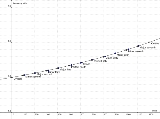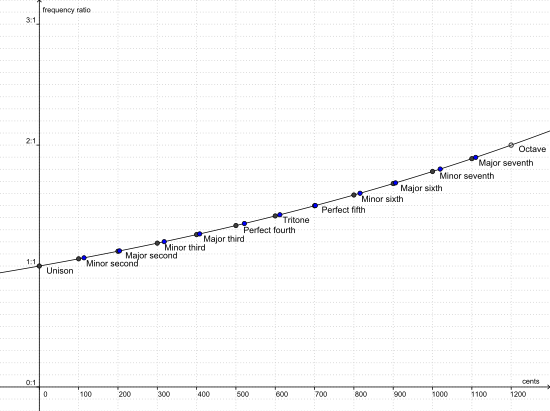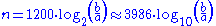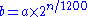xCent (music)Encyclopedia
The cent is a logarithmic
Logarithmic scale
A logarithmic scale is a scale of measurement using the logarithm of a physical quantity instead of the quantity itself.A simple example is a chart whose vertical axis increments are labeled 1, 10, 100, 1000, instead of 1, 2, 3, 4...

unit of measure used for musical intervals
Interval (music)
In music theory, an interval is a combination of two notes, or the ratio between their frequencies. Two-note combinations are also called dyads...

. Twelve-tone equal temperament
Equal temperament
An equal temperament is a musical temperament, or a system of tuning, in which every pair of adjacent notes has an identical frequency ratio. As pitch is perceived roughly as the logarithm of frequency, this means that the perceived "distance" from every note to its nearest neighbor is the same for...

divides the octave
Octave
In music, an octave is the interval between one musical pitch and another with half or double its frequency. The octave relationship is a natural phenomenon that has been referred to as the "basic miracle of music", the use of which is "common in most musical systems"...

into 12 semitones of 100 cents each. Typically, cents are used to measure extremely small finite intervals, or to compare the sizes of comparable intervals in different tuning systems, and in fact the interval of one cent is much too small to be heard between successive notes.

Alexander J. Ellis based the measure on the acoustic logarithms decimal semitone system developed by Gaspard de Prony
Gaspard de Prony
Gaspard Clair François Marie Riche de Prony was a French mathematician and engineer, who worked on hydraulics. He was born at Chamelet, Beaujolais, France and died in Asnières-sur-Seine, France.-Education and early works:...

in the 1830s, at Robert Holford Macdowell Bosanquet's suggestion. Ellis made extensive measurements of musical instruments from around the world, using cents extensively to report and compare the scales employed, and further described and employed the system in his edition of Hermann von Helmholtz
Hermann von Helmholtz
Hermann Ludwig Ferdinand von Helmholtz was a German physician and physicist who made significant contributions to several widely varied areas of modern science...

's On the Sensations of Tone. It has become the standard method of representing and comparing musical pitches and intervals with relative accuracy.

## UseLike a decibel
Decibel
The decibel is a logarithmic unit that indicates the ratio of a physical quantity relative to a specified or implied reference level. A ratio in decibels is ten times the logarithm to base 10 of the ratio of two power quantities...

's relation to intensity, a cent is a ratio between two close frequencies. For the ratio to remain constant over the frequency spectrum, the frequency range encompassed by a cent must be proportional to the two frequencies. An equally tempered
Equal temperament
An equal temperament is a musical temperament, or a system of tuning, in which every pair of adjacent notes has an identical frequency ratio. As pitch is perceived roughly as the logarithm of frequency, this means that the perceived "distance" from every note to its nearest neighbor is the same for...

semitone
Semitone
A semitone, also called a half step or a half tone, is the smallest musical interval commonly used in Western tonal music, and it is considered the most dissonant when sounded harmonically....

(the interval between two adjacent piano keys) decidedly spans 100 cents. An octave
Octave
In music, an octave is the interval between one musical pitch and another with half or double its frequency. The octave relationship is a natural phenomenon that has been referred to as the "basic miracle of music", the use of which is "common in most musical systems"...

-- two notes that have a fequency ratio of 2:1 -- spans twelve semitones and therefore 1200 cents. Since a frequency raised by one cent is simply multiplied by this constant cent value, and 1200 cents doubles a frequency, the ratio of frequencies one cent apart is precisely equal to 21/1200, the 1200th root of 2, which is approximately 1.0005777895.

If one knows the frequencies a and b of two notes, the number of cents measuring the interval from a to b may be calculated by the following formula (similar to the definition of decibel):Likewise, if one knows a note a and the number n of cents in the interval from a to b, then b may be calculated by:To compare different tuning systems, convert the various interval sizes into cents. For example, in just intonation
Just intonation
In music, just intonation is any musical tuning in which the frequencies of notes are related by ratios of small whole numbers. Any interval tuned in this way is called a just interval. The two notes in any just interval are members of the same harmonic series...

the major third is represented by the frequency ratio 5:4. Applying the formula at the top shows this to be about 386 cents. The equivalent interval on the equal-tempered piano would be 400 cents. The difference, 14 cents, is about a seventh of a half step, easily audible. The just noticeable difference
Just noticeable difference
In psychophysics, a just noticeable difference, customarily abbreviated with lowercase letters as jnd, is the smallest detectable difference between a starting and secondary level of a particular sensory stimulus...

for this unit is about 6 cents.

## Piecewise linear approximation

As x increases from 0 to 1/12, the function 2x increases almost linearly from 1.00000 to 1.05946. The exponential cent scale can therefore be accurately approximated as a piecewise linear function which is exact at semitones and whose maximum error occurs halfway between semitones. That is, n cents for n from 0 to 100 may be approximated as 1+.0005946n instead of 2n/1200. The error is zero when n is 0 or 100, and is about 0.72 cents high when n is 50, where the correct value of 21/24 = 1.02930 is approximated by 1 + .0005946*50 = 1.02973. This error is well below anything humanly audible, making this piecewise linear approximation suitable for most practical purposes while being conceptually and numerically easier to work with in many situations.

## Human perception

It is difficult to establish how many cents are perceptible to humans; this accuracy varies greatly from person to person. One author stated that humans can distinguish a difference in pitch of about 5-6 cents. The threshold of what is perceptible, technically known as the just noticeable difference
Just noticeable difference
In psychophysics, a just noticeable difference, customarily abbreviated with lowercase letters as jnd, is the smallest detectable difference between a starting and secondary level of a particular sensory stimulus...

, also varies as a function of the timbre
Timbre
In music, timbre is the quality of a musical note or sound or tone that distinguishes different types of sound production, such as voices and musical instruments, such as string instruments, wind instruments, and percussion instruments. The physical characteristics of sound that determine the...

of the pitch: in one study, changes in tone quality reduced student musicians' ability to recognize as out-of-tune pitches that deviated from their appropriate values by ±12 cents. It has also been established that increased tonal context enables listeners to judge pitch more accurately.

When listening to pitches with vibrato
Vibrato
Vibrato is a musical effect consisting of a regular, pulsating change of pitch. It is used to add expression to vocal and instrumental music. Vibrato is typically characterised in terms of two factors: the amount of pitch variation and the speed with which the pitch is varied .-Vibrato and...

, there is evidence that humans perceive the mean frequency as the center of the pitch. One study of vibrato in western vocal music found a variation in cents of vibrato typically ranged between ±34 cents and ±123 cents, with a mean variation of ±71 cents; the variation was much higher on Verdi opera arias.

Normal adults are able to recognize pitch differences of as small as 25 cents very reliably. Adults with amusia
Amusia
Amusia is a musical disorder that appears mainly as a defect in processing pitch, but it also encompasses musical memory and recognition. Two main classifications of amusia exist: acquired amusia, which occurs as a result of brain damage, and congenital amusia, which results from a music processing...

, however, have trouble recognizing differences of less than 100 cents and sometimes have trouble with these or larger intervals.

## Sound files

The following audio files play various intervals. In each case the first note played is middle C. The next note is sharper than C by the assigned value in cents. Finally, the two notes are played simultaneously.

Played separately, the notes may not show an audible difference, but when they are played together, a beat
Beat (acoustics)
In acoustics, a beat is an interference between two sounds of slightly different frequencies, perceived as periodic variations in volume whose rate is the difference between the two frequencies....

may be heard. At any particular instant, the two waveforms reinforce or cancel each other more or less, depending on their instantaneous phase
Phase (waves)
Phase in waves is the fraction of a wave cycle which has elapsed relative to an arbitrary point.-Formula:The phase of an oscillation or wave refers to a sinusoidal function such as the following:...

relationship. A piano tuner may verify tuning accuracy by timing the beats when two strings are sounded at once.